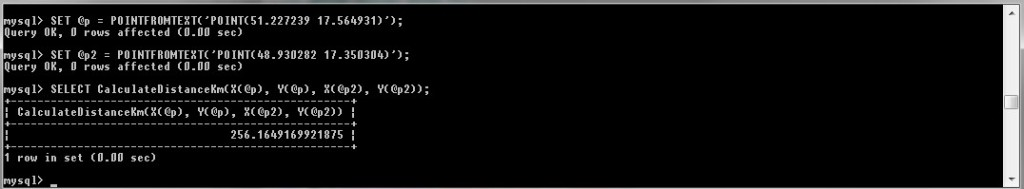© 2013 All rights reserved.

# MySQL: Working with coordinates in the database

How to insert coordinates in the database and how to operate with them in MySQL.For all examples i have this two simple tables. Both tables contains 3000 rows with data:

The first table has a column ready to save the coordinate values separately, the second table will store the coordinates as POINT.

## Save coordinates separately

This way we all know, we store the longitude and latitude separately with simple sql query:

To obtain data we can use a simple query like the following:

This is not anything new and interesting.

## Store the coordinates as POINT

The possibility that I would like to show is save the coordinates in point form. A Point is a geometry that represents a single location in coordinate space.

Work of this type is simple. This is a simple example:

This code declare local variable in Mysql and select data from this variable. Variable is declared as POINT type, and contain coordinates.
In this case we can simple get X and Y coordinates from variable as you can see.

In the database we can save this variable simply as follows:

And again select values from table:

The result will look like:

ASWKT – Converts a value in internal geometry format to its WKT representation and returns the string result.
Again, we can easily access to the items saved in the coordinates with X and Y “axes”.

### Distance between the points

In this case when we have values ​​stored as POINT, we can use the operations of this type variable. As calculate distance between two points. This is not a geometric distance, but only the distance in the polygon points.

As a result we get the distance value in units. If we calculate the distance between two points that differ by one unit, we would get a value of 1.

If we want to calculate the distance, we would have a saved value of the distance from the central point.

## Calculation of the distance from a given point

If you want to calculate the distance from a certain point, we can proceed in both cases as well.

### Coordinates are separately

Calculate the distance between two points for each row:

### Coordinates are POINT format

As in the previous example, only approaching the coordinates through the values from POINT variable:

### Create a function to calculate the distance

Pouze doporučení: V tomto případě je lepší vytvořit jednoduchou funkci v databázi.

Now we can use the same in both cases:

## in conclusion

Neither way is probably better, it always depends on the use of.

## Comments (7)Thank you for this articlenabil

Very nice article.I have many gps trajectory data, it have 4 row for id, date time, lat, long. How to calculate all of data to find the distance from start and last trajectory using Haversine or other query? I don’t know to set the lat1, lat2, lon1, lo2 if the data is so big.XWAmazing! Its truly amazing article, I have got much clear idea regarding from this article.Hello,Thanks for such a great informative article…But i have a question how can i get results within (lat1,long1) and (lat2,long2) so that i can get list of all the location from db …The db have id,lat,long,name,country,user_count

Regardssuch a great article . really saved my day

### Trackbacks for this post

About
Hi, i am programmer from the Czech Republic. I love web development (Ruby, Ruby on Rails, PHP, Nette) and iOS development (Objective-C, Cocoa).
To cooperate, here is my phone:
+420 608 836